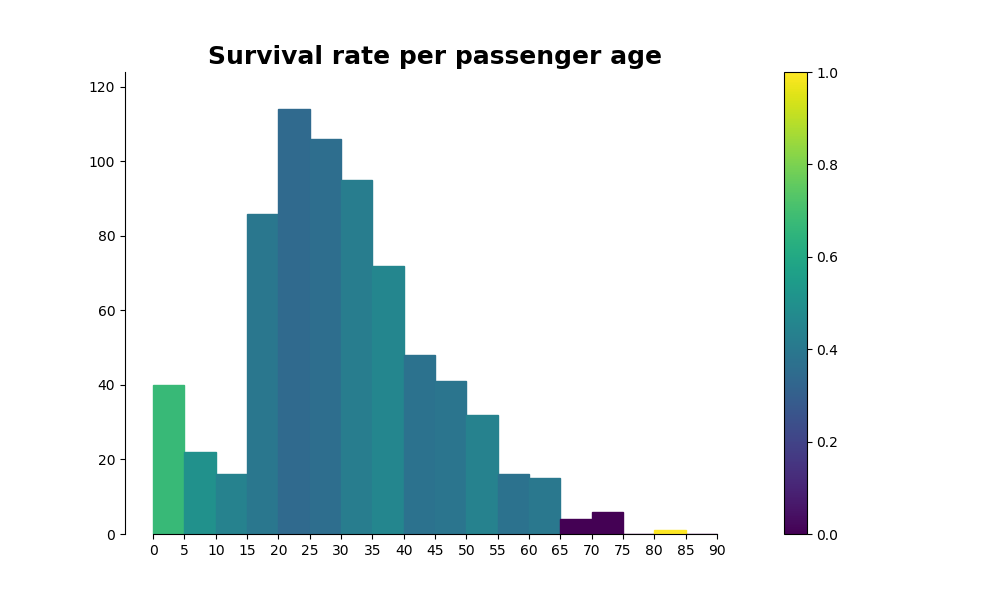Since I started studying Python for data science, I have used Matplotlib for data visualization and found the library quite confusing.
When I search for help on internet, I see different ways to do the same things. For example, to draw bars, some will use `plt.bar()` and others `ax.bar()`.
Things are getting worst when you try to customize your graph (i.e. use subplots, modify axis, ...).

The reason for this diversity of approaches to set a plot is that there are two API in Matplotlib. The `pyplot` API and an `object-oriented` API. It is advised to learn the `object-oriented` API and I'll try to stick to it as much as I can in this post.

The seaborn library can help us create nice graphics easily, but when we want to display multiple charts at once or tweak the look of the charts, we also need to be able to work with matplotlib API.

I'll use the titanic dataset to create some of the charts. It can be downloaded from Kaggle.

Let's get started! First thing, import matplotlib.

``````import matplotlib
``````

## Backend

Matplotlib has many use cases. It can be used from the python shell or in jupyter notebooks or it can be embeded in applications interface.
We can choose from different backends to handle all those different cases.

There are two types of backends: - user interface backends or interactive backends to display on GUI. - hardcopy backends or non-interactive backends to generate image files.

The backend has to be set before we import pyplot.

There are two ways to set the backend: `matplotlib.use('backend_name')` or `%matplotlib backend_name`.
The second method is an iPython magic command and will not work in all circumstances.

``````# View what is the current selected backend.
matplotlib.get_backend()
``````
``````'module://ipykernel.pylab.backend_inline'
``````

We can easily get a list of available backends

``````print('interactive backends:\n{}\n'.format(matplotlib.rcsetup.interactive_bk))
print('non interactive backends:\n{}\n'.format(matplotlib.rcsetup.non_interactive_bk))
print('all backends: \n{}\n'.format(matplotlib.rcsetup.all_backends))
``````
``````interactive backends:
['GTK', 'GTKAgg', 'GTKCairo', 'MacOSX', 'Qt4Agg', 'Qt5Agg', 'TkAgg', 'WX', 'WXAgg', 'GTK3Cairo', 'GTK3Agg', 'WebAgg', 'nbAgg']

non interactive backends:
['agg', 'cairo', 'gdk', 'pdf', 'pgf', 'ps', 'svg', 'template']

all backends:
['GTK', 'GTKAgg', 'GTKCairo', 'MacOSX', 'Qt4Agg', 'Qt5Agg', 'TkAgg', 'WX', 'WXAgg', 'GTK3Cairo', 'GTK3Agg', 'WebAgg', 'nbAgg', 'agg', 'cairo', 'gdk', 'pdf', 'pgf', 'ps', 'svg', 'template']
``````

Here, I'll use the nbAgg backend.

``````matplotlib.use('nbAgg')
matplotlib.get_backend()
``````
``````'nbAgg'
``````

now that the backend is set, we can import pyplot

``````import matplotlib.pyplot as plt
``````

## Figure

The `figure` is the top level container that will contain everything we draw.
When creating a figure, we can specify its size using the `figsize` parameter. `figsize` is a width, height tuple.

``````    # Create a figure with width = 8 and height = 5.
fig = plt.figure(figsize=(8, 5))
``````

We can also use the `figaspect` function to set the aspect ratio of our figure:

``````    # Create a figure that is twice as tall as it is wide.
fig = plt.figure(figsize=plt.figaspect(2.0))
``````

## Axes

`Axes` are the area in which the data is plotted. A `Figure` can have multiple `Axes` but `Axes` belong to one `Figure` only.
We can add `Axes` to a `Figure` using the `add_axes()` or `add_subplot()` methods. `Subplots` and `Axes` are the same thing.
`add_axes()` takes the `rect` parameter. `rect` is a sequence of floats that specifies [left, bottom, width, heights]. So the `Axes` are positioned in absolute coordinates.

``````    ax = fig.add_axes([0,0,1,1])
``````

`add_subplots()` takes 3 integers as parameter. Those 3 numbers set the number of rows and columns and the position of the subplot in the grid: `add_subplots(ijk)` add an `Axes` in the kth position of a grid that has i rows and j columns.
`add_subplots()` is the easiest way to setup your layout while `add_axes()` will give you more control over the position of your `Axes`.

``````    # Create a new axes at the first position of a 2 rows by 3 columns grid.
ax = fig.add_subplot(231)
``````

### single Axes

``````# Create a figure.
fig = plt.figure(figsize=(6, 4))

# Add an axes.
ax = fig.add_subplot(111)

plt.show()
``````### Multiple subplots

We can create a figure with multiple subplots by calling `add_subplot()` for each subplot we want to create.
Here's an example for a 2 by 2 layout:

``````fig = plt.figure(figsize=(6, 4))

ax1 = fig.add_subplot(221)
ax1.set_title('first subplot')

ax2 = fig.add_subplot(222)
ax2.set_title('second subplot')

ax3 = fig.add_subplot(223)
ax3.set_title('third subplot')

ax4 = fig.add_subplot(224)
ax4.set_title('fourth subplot')

fig.tight_layout()
plt.show()
``````This method is not the most efficient, especially if we want to draw a lot of subplots.
An alternative way is to use the `plt.subplots()` function.
The function returns a figure and an array of axes.

``````fig, axes = plt.subplots(nrows=2, ncols=2)

# We can now access any Axes the same way we would access
# an element of a 2D array.
axes[0,0].set_title('first subplot')
axes[0,1].set_title('second subplot')
axes[1,0].set_title('third subplot')
axes[1,1].set_title('fourth subplot')

fig.tight_layout()
plt.show()
``````Another way to create grid layouts is to use the `gridspec` module. It lets us specify the location of subplots in the figure. It also makes it easy to have plots that span over multiple columns

``````import matplotlib.gridspec as gridspec

fig = plt.figure()

# I use gridspec de set the grid.
# I need a 2x2 grid.
G = gridspec.GridSpec(2, 2)

# The first subplots is on the first row and span over all columns.
ax1 = plt.subplot(G[0, :])

# The second subplot is on the first column of the second row.
ax2 = plt.subplot(G[1, 0])

# The third subplot is on the second column of the second row.
ax3 = plt.subplot(G[1, 1])

fig.tight_layout()
plt.show()
``````Or we can have a subplot that spans over multiple rows

``````fig = plt.figure()

# The first subplot is two rows high.
ax1 = plt.subplot(G[:, :1])

# The second subplot is on the second column of the first row.
ax2 = plt.subplot(G[0, 1])

# The third subplot is on the second column of the second row.
ax3 = plt.subplot(G[1, 1])

fig.tight_layout()
plt.show()
``````Using `gridspec`, we can also have different sizes for each subplot by specifying ratios for width and heights.

``````fig = plt.figure()

G = gridspec.GridSpec(2, 2,
width_ratios=[1, 2], # The second column is two times larger than the first one.
height_ratios=[4, 1] # The first row is four times higher than the second one.
)

# In this example, I use a different way to refer to a grid element.
# Note that it is not clear in which part of the grid the subplot is.
ax1 = plt.subplot(G) # same as plt.subplot(G[0, 0])
ax2 = plt.subplot(G) # same as plt.subplot(G[0, 1])
ax3 = plt.subplot(G) # same as plt.subplot(G[1, 0])
ax4 = plt.subplot(G) # same as plt.subplot(G[1, 1])

fig.tight_layout()
plt.show()
``````## Artists

`Artists` are everything we can see on a `Figure`. Most of them are tied to an `Axes`.

## Plotting

I won't spend to much time on the ploting functions. Everybody has already used them extensively and there are a lot of tutorials out there.
I'll just add that the plotting functions we usually call using pyplot (e.g. `plt.scatter()`) can be called on Axes: `ax.scatter()`. This make it easy to manage multiple subplots

``````fig, ax = plt.subplots(figsize=(6, 4), nrows=1, ncols=2)

# Plot different charts on each axes.
ax.bar(np.arange(0, 3), df["Embarked"].value_counts())
ax.bar(np.arange(0, 3), df["Pclass"].value_counts())

# Customize a bit.
ax.set_title('Embarked')
ax.set_title('Pclass')

fig.tight_layout()
plt.show()
``````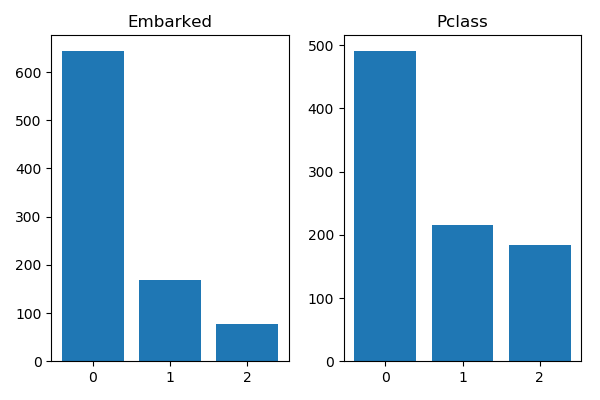## Customization

Now that we have set the layout and plot some data, we can have a look at how to customize the elements or `Artists` of our plot.

### Axis and Ticks

`Axis` are the X and Y axis of our plot, not to be confused with the `Axes`.
Each `Axis` has `Ticks` that can also be customized. Let's look at a sample plot.

``````x = np.arange(0, 10, 0.1)
y = x**2
fig = plt.figure()
ax = fig.add_subplot(111)
ax.plot(x, y)
plt.show()
``````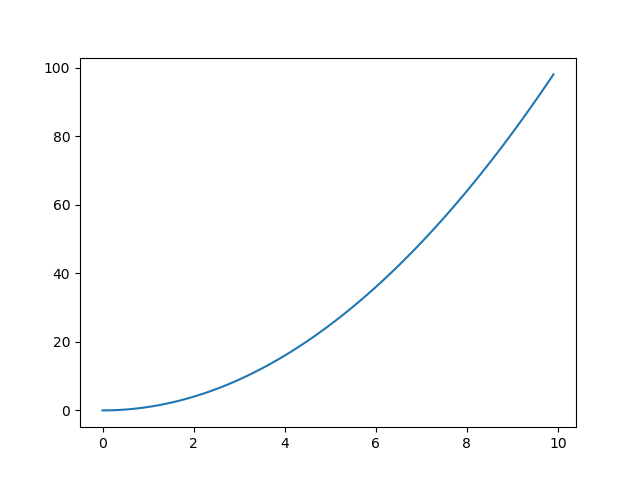The `Ticks` on the X-axis are the marks with the numbers 0 to 10 and the ticks on the Y-axis are the marks with numbers 0 to 100.
Customizing the axis
Some useful customizations on the axis are:

• `ax.set_xlabel()`, `ax.set_ylabel()`: add a label to the axis
• `ax.set_xlim()`, `ax.set_ylim()`: to set the data limits on the axis. We can use `get_xlim()` or `get_ylim()` to see what are those limits.

Customizing the ticks We can customize the `Ticks` using the `tick_params()` method.
The most useful options are: - bottom, top, left, right: set to True/False or 'on'/'off' to show or hide the ticks - labelbottom, labeltop, labelleft, labelright: set to True/False or 'on'/'off' to show or hide the ticks labels - labelrotation: rotate the tick labels - labelsize: resize the ticks labels - labelolor: change the color of ticks labels - color: change the ticks colors

``````fig = plt.figure()
ax = fig.add_subplot(111)
ax.plot(x, y)

# Add labels.
# We can also use LaTex notations in titles and labels.
ax.set_xlabel('\$x\$')
ax.set_ylabel('\$y = x^2\$')

# Reduce the axis limites to let the line touch the borders.
ax.set_xlim(0, 10)
ax.set_ylim(0, 100)

# Customize the ticks.
ax.tick_params(labelleft=False,
labelcolor='orange',
labelsize=12,
bottom=False,
color='green',
width=4,
length=8,
direction='inout'
)

plt.show()
``````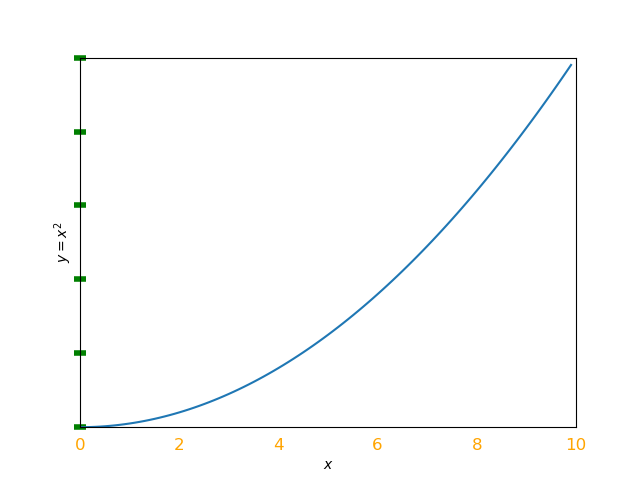### Spines

The spines are the lines that surround your plot. Two of them define the X and Y axis. The two others close the frame.
We can set bounds to limit the spine length and show or hide a spine.

``````fig = plt.figure()
ax = fig.add_subplot(111)

# Shorten the spines used for the x and y axis.
ax.spines['bottom'].set_bounds(0.2, 0.8)
ax.spines['left'].set_bounds(0.2, 0.8)

# Other customizations.
ax.spines['bottom'].set_color('r')
ax.spines['bottom'].set_linewidth(2)

# Remove the two other spinces
ax.spines['top'].set_visible(False)
ax.spines['right'].set_visible(False)

plt.show()
``````### Bars, lines, markers, ...

Plotting functions return objects. For example `ax.bar(x, y)` returns a container with all the bars. We can catch the bars and call methods on them.
We can find the methods in the matplotlib documentation.

We can get the bars attributes: - get_height() - get_width() - get_x(), get_y(), get_xy()

or set a new value for those attributes: - set_height() - set_width() - set_x(), set_y(), set_xy() - set_color() - ...

``````# Lets create some bars.
x = np.arange(0, 5)
y = [2, 6, 7, 3, 4]
bars = plt.bar(x, y, color='b')

# We can get the height of the bars.
for i, bar in enumerate(bars):
print('bars[{}]\'s height = {}'.format(i, bar.get_height()))

# Or we can set a different color for the third bar.
bars.set_color('r')

# Or set a different width for the first bar.
bars.set_width(0.4)

plt.show()
``````
``````bars's height = 2
bars's height = 6
bars's height = 7
bars's height = 3
bars's height = 4
``````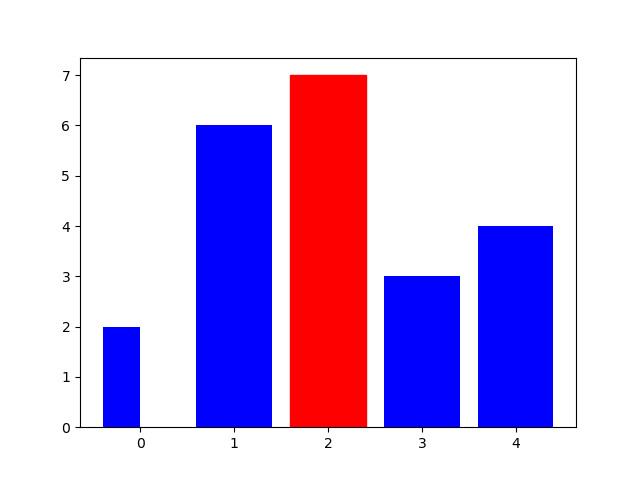The `hist()` method returns an array of the bins values, an array of bins and the patches used to draw the histogram. In the following example, I'll look at the age distribution of the Titanic's passengers. I'll set a different color to the highest bar to have it stand out.

``````fig = plt.figure()
ax = fig.add_subplot(111)

x = df['Age'].dropna()
bins = np.arange(0, 95, 5)
values, bins, bars = ax.hist(x, bins=bins)

# Get the value of each bin.
for bin, value in zip(bins, values):
print('bin {}: {} passengers'.format(bin, int(value)))

# Change the highest bin color.
max_idx = values.argmax()
bars[max_idx].set_color('r')

plt.show()
``````
``````bin 0: 40 passengers
bin 5: 22 passengers
bin 10: 16 passengers
bin 15: 86 passengers
bin 20: 114 passengers
bin 25: 106 passengers
bin 30: 95 passengers
bin 35: 72 passengers
bin 40: 48 passengers
bin 45: 41 passengers
bin 50: 32 passengers
bin 55: 16 passengers
bin 60: 15 passengers
bin 65: 4 passengers
bin 70: 6 passengers
bin 75: 0 passengers
bin 80: 1 passengers
bin 85: 0 passengers
``````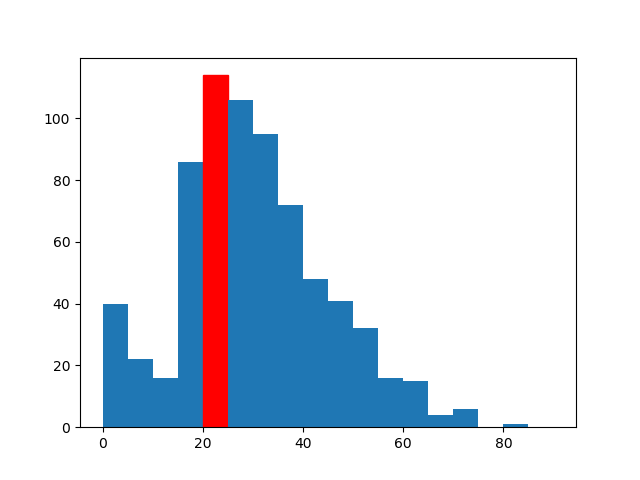### Annotations

We can add text to our plot with `ax.annotation()` and `ax.text()`.
`ax.annotation('text', (x, y)`
`ax.text(x, y, 'text', **kwargs)`

If needed, we can add arrows to point our annotation to a specific point. Just use `ax.arrow(x, y, dx, dy)` where `x` and `y` are the coordinates of the origin of the arrow and `dx`, `dy` are the length of the arrow along the coordinates.
We can also add more arguments to customize the arrow: - width: width of the arrow tail - head_width: width of the arrow head - head_length: length of the arrow head - shape: shape of the arrow head ('full', 'left' or 'right') - edgecolor, facecolor - color (will override edgecolor and facecolor) - ...

Let's look at an example.

``````x = np.arange(0, 10, 0.1)
y = x**2
fig = plt.figure()
ax = fig.add_subplot(111)
ax.plot(x, y)

ax.annotate('bar', (5, 20))

ax.text(1,
50,
'foo',
fontsize=14,
color='red')

# Add an arrow that starts at 'foo' and point at the line.
ax.arrow(1.5, 48,
0.5, -44,
length_includes_head=True,
width=0.3,
head_length=4,
facecolor='y',
edgecolor='r',
shape='left')

# We can also set an arrow in the annotate method.
ax.annotate('quz',
xytext=(6, 60), # text coordinates
xy=(7.8, 60),   # arrow head coordinates
arrowprops=dict(arrowstyle="->"))

plt.show()
``````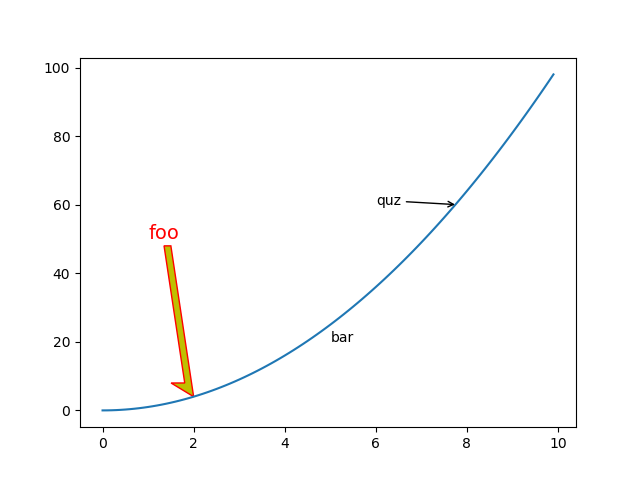## Practice

Now, let's use everything we've learn to create some nice visualizations.

### Exercise 1

In this first exercise, I am going to improve a simple bar chart showing the number of passengers per port of embarkation.
Here's the default chart rendered by pyplot.

``````fig = plt.figure()
ax = fig.add_subplot(111)
ax.bar(np.arange(0, 3), df["Embarked"].value_counts())
plt.show()
``````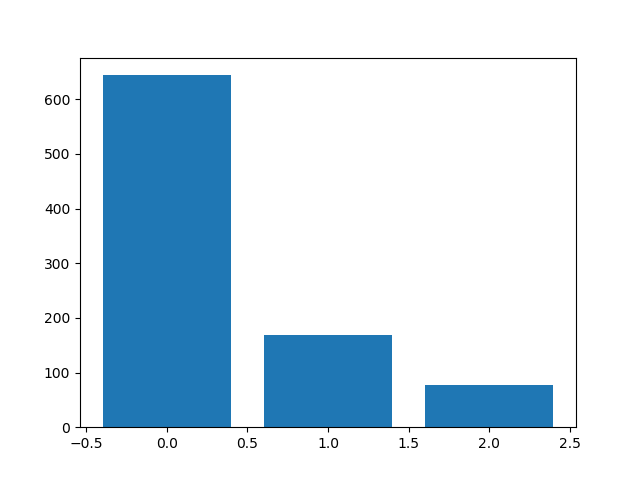I am going to remove unecessary elements and change the colors.
I'll add some meaningful information like labels and values on bars and a title.

``````fig = plt.figure(figsize=(8, 6))
ax = fig.add_subplot(111)

x = np.arange(0, 3)
y = df['Embarked'].value_counts()
bars = ax.bar(x, y, color='lightslategrey')

# Remove the frame.
ax.set_frame_on(False)

# We need only 3 ticks (one per bar).
ax.set_xticks(np.arange(0, 3))

# We don't want the ticks, only the labels.
ax.tick_params(bottom='off')
ax.set_xticklabels(['Southampton', 'Cherbourg', 'Queenstown'],
{'fontsize': 12,
'verticalalignment': 'center',
'horizontalalignment': 'center',
})

# Remove ticks on the y axis and show values in the bars.
ax.tick_params(left='off',
labelleft='off')

# Add the values on each bar.
for bar, value in zip(bars, y):
ax.text(bar.get_x() + bar.get_width() / 2, # x coordinate
bar.get_height() - 5,              # y coordinate
value,                             # text
ha='center',                       # horizontal alignment
va='top',                          # vertical alignment
color='w',                         # text color
fontsize=14)

# Use a different color for the first bar.
bars.set_color("firebrick")

# Add a title.
ax.set_title('Most of passengers embarked at Southampton',
{'fontsize': 18,
'fontweight' : 'bold',
'verticalalignment': 'baseline',
'horizontalalignment': 'center'})

plt.show()
``````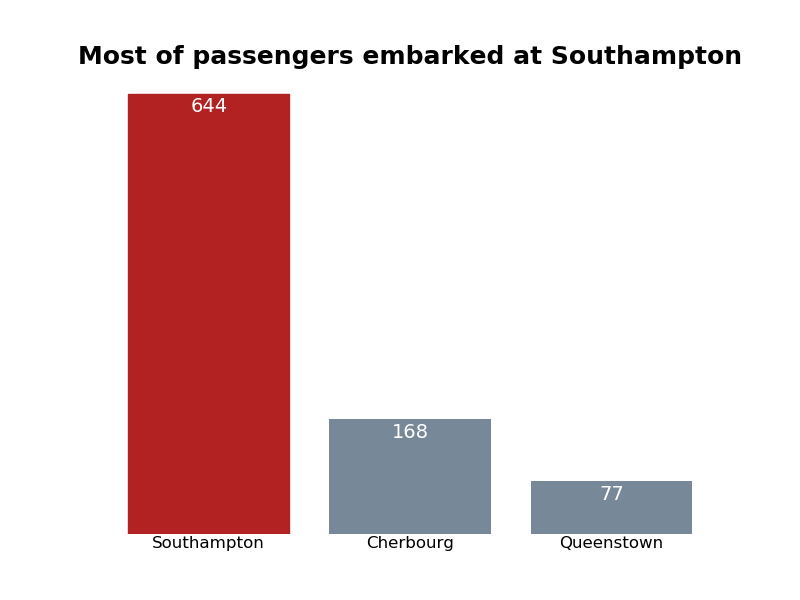### Exercise 2

In this second exercise, I am going to plot the distribution of passengers per age and color the bars depending on survival rate.

``````fig = plt.figure(figsize=(10, 6))
ax = fig.add_subplot(111)

x = df['Age'].dropna()
age_bins = np.arange(0, 95, 5)
values, bins, bars = ax.hist(x, bins=age_bins)

ax.set_xticks(bins)

ax.set_ylim(values.min(), values.max() + 10)

ax.spines['bottom'].set_bounds(bins.min(), bins.max())
ax.spines['right'].set_bounds(values.min(), values.max())

ax.spines['top'].set_visible(False)
ax.spines['right'].set_visible(False)

cm = plt.cm.get_cmap('viridis')

for i in range(0, len(bins)):
if i < len(bins) - 1:
survival = df[(df.Age >= bins[i]) & (df.Age < bins[i + 1])]['Survived'].mean()
else:
survival = df[(df.Age >= bins[i])]['Survived'].mean()
try:
bars[i].set_color(cm(survival))
except:
pass

# Add colorbar.
# The survival rate is already normalized so we don't need norm=plt.Normalize(vmin=0, vmax=1).
# I left it as an example.
sm = plt.cm.ScalarMappable(cmap=cm, norm=plt.Normalize(vmin=0, vmax=1))
sm._A = []
plt.colorbar(sm)

# Add a title.
ax.set_title('Survival rate per passenger age',
{'fontsize': 18,
'fontweight' : 'bold',
'verticalalignment': 'baseline',
'horizontalalignment': 'center'})

plt.show()
``````